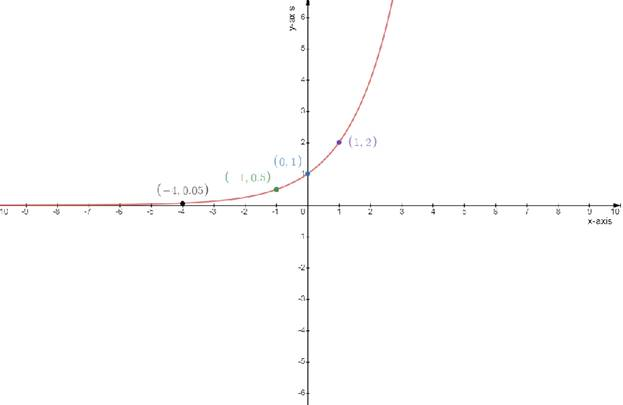# The graph of f has a vertical tangent or a vertical cusp at c### Single Variable Calculus: Concepts...

4th Edition
James Stewart
Publisher: Cengage Learning
ISBN: 9781337687805### Single Variable Calculus: Concepts...

4th Edition
James Stewart
Publisher: Cengage Learning
ISBN: 9781337687805

#### Solutions

Chapter 2.2, Problem 28E
To determine

## To find:The graph of f has a vertical tangent or a vertical cusp at c

Expert Solution

f'(1)=

### Explanation of Solution

Given:

The function f(x)=2x;(0,1)

Concept used:

The definition of a vertical cusp is that the one sided limits of the derivative approach opposite ± : positive infinity on one side and negative infinity on the other side . A vertical tangent has the one sided limits of the derivative equal to the same sign of infinity

The derivative at the relevant point is undefined in both the cusp and the vertical tangent Calculation:

The function is f(x)=2x;(0,1).........(1)

The first derivative and chain rule

f'(x)=2x1x

To draw a table

The vertical tangent means that the derivative at that point approaches infinity

Since the slope is infinitely large

Test one point in each of the region formed by the graph

If the point satisfies the function then shade the entire region to denote that every point in the region satisfies the function .

 x−axis 0 −1 −4 1 y−axis 1 0.5 0.05 2

To draw a graph### Have a homework question?

Subscribe to bartleby learn! Ask subject matter experts 30 homework questions each month. Plus, you’ll have access to millions of step-by-step textbook answers!